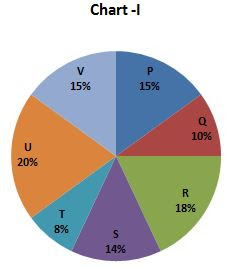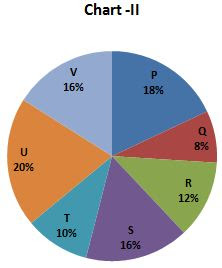# Quantitative Aptitude Practice Questions for Bank Exams, IPPB and RBI Assistant

## Quantitative Aptitude Practice Questions for Bank Exams, IPPB and RBI Assistant

Directions (1 – 5): Study the following graph carefully and answer the questions given below:
The following Pie- charts show the distribution of candidates enrolled for Engineering (Chart-I) and the candidates who have successfully completed the course (Chart-II) from different institutes.
Candidates enrolled = 12000Candidates who have successfully completed the course = 90001. Which institute has the highest percentage of students successful with respect to those enrolled?
a) V
b) U
c) R
d) S
e) None of these
2. What percent of candidates from institutes T have come out to be successful out of the total candidates enrolled in the institutes?
a) 60%
b) 75%
c) 45%
d) 55%
e) None of these
3. In which of the following institutes, the number of successful candidates is equal to the number of enrolled candidates in institute S?
a) P
b) Q
c) R
d) T
e) None of these
4. What is the ratio candidates successful to those enrolled for institute U and V together?
a) 45 : 46
b) 35 : 48
c) 33 : 47
d) 35 : 42
e) None of these
5. If there were 15% candidates enrolled in institute S in place of 16%, then what would be the success percentage in institute S?
a) 20%
b) 40%
c) 60%
d) 70%
e) None of these
Directions (6 – 10): In each of these questions, two equations (I) and (II) are given. You have to solve both the equations and give answer:
a) if x > y
b) if x ≥ y
c) if x < y
d) if x ≤ y
e) if x = y or relationship cannot be established
6. I. 5x2 - 29x + 20= 0 II. 6y2 - 23y+7 =  0
7. I. x = 3√6859 II. y2 = 361
8. I.x2 - 6x - 27=0 II.y2+9y+20=0
9. I.2x2 + 19x +42 = 0 II. 4y2 +43y+30=0
10. I.x2-30x+200=0 II.3y2-40y+125=0
Solutions:
1. C) Here, we use simply divide the % number of successful candidatesthe by the % number of candidates enrolled in the same institute then we got R has maximum percentage.
2. A) Required Percentage = (9000*8%)/(12000*10%) *100 = 720/1200 *100 = 60 %
3. E) No any institute of successful candidates is equal to the number of enrolled candidates in institutes S
4. B) Total successful candidates for institute U and V = (9000*35)/100 = 3150
Total enrolled candidates for institute U and V = (12000*36)/100 = 4320
Required ratio = 3150/4320 = 35 : 48
5. D) The success percentage in Institute S = (9000*14%)/(12000*15%) *100 = 70%
6. E) I.5x2 - 29x+20=0
(5x-4) (x-5)
x = +4/5, +5
II. 6y2 - 23y+7 = 0
(3y-1) (2y-7)
y = +1/3, +7/2
7. B) x = 19; y = ± 19 so x ≥ y
8. A) I.x2 - 6x - 27=0
(x+3) (x-9)
x = -3, +9
II.y2+9y+20=0
(y+4) (y+5)
y = -4, -5
9. E) I. 2x2 + 19x +42 = 0
(2x+7) (x+6)
x = -7/2, -6
II. 4y2 +43y+30=0
(4y+3) (y+10)
y = -3/4, -10